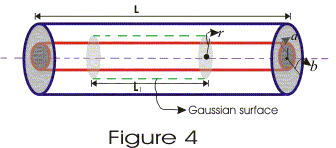# Cylinderical capacitor

## Cylinderical capacitor

• A cylinderical capacitor is made up of a conducting cylinder or wire of radius a surrounded by another concentric cylinderical shell of radius b (b>a).

• Let L be the length of both the cylinders and charge on inner cylender is +Q and charge on outer cylinder is -Q.
• For calculate electric field between the conductors using Gauss's law consider a Gaussian surface of radius r and length L1 as shown in figure 4.• According to Gauss's law flux through this surface is $\frac {q}{\epsilon _0}$ where q is net charge inside this surface.
• We know that electric flux is given by
$\phi =E.A$
$=EA cos \theta$
$=EA$
since electric field is constant in magnitude on the Gaussian surface and is perpendicular to this surface. Thus,
$\phi=E(2 \pi rL)$
since $\phi=\frac {q}{\epsilon _0}$
=> $E(2 \pi rL)=\frac {\lambda L}{\epsilon _0}$
where $\lambda = Q/L$ = charge per unit length
So, $E=\frac{\lambda }{2\pi \epsilon _{0}r}$
• If potential at inner cylinder is Va and Vb is potential of outer cylinder then potential difference between both the cylinders is
V=Va and Vb=∫Edr
where limits of integration goes from a to b.

• Potential of inner conductor is greater then that of outer conductor because inner cylinder carries positive charge. Thus potential difference is $$V=\frac {Qln(b/a)}{2\pi \epsilon _{0}L}$$
• Thus capacitance of cylinderical capacitor is
$C=\frac {Q}{V} or,$C=\frac{2\pi \epsilon _{0}L}{ln (b/a)}$------(5) • From equation 5 it can easily be concluded that capacitance of a cylinderical capacitor depends on length of cylinders. • More is the length of cylinders , more charge could be stored on the capacitor for a given potential difference. Question A cylindrical capacitor is constructed using two coaxial cylinders of the same length 10 cm of radii 5 mm and 10 mm. (a) calculate the capacitance (b) another capacitor of the same length is constructed with cylinders of radii 8 mm and 16 mm. Calculate the capacitance . Solution Capacitance is given by$C=\frac{2\pi \epsilon _{0}L}{ln (b/a))}$(a) Here L=10 cm = .1 m b=10 mm=, a=5 mm$C= \frac {2 \times 3.14 \times 8.88 \times 10^{-12} \times .10}{ln (10/5}=8 pF\$
(b) Now b=16 mm and a=8 mm
Since ln 16/8 = ln 10/5
C= 8pF